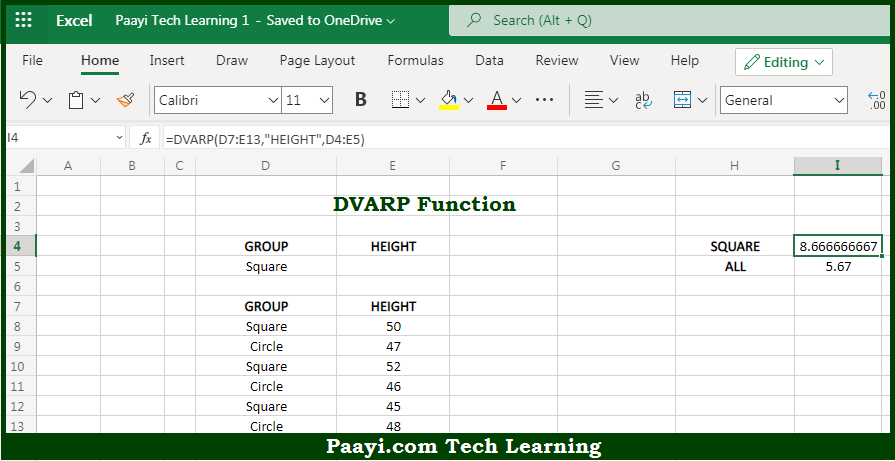# Learn How to Use Microsoft Excel DVARP Function

Written by | 0 Comments | 611 Views

In this article, you will learn how to use the Microsoft Excel DVARP function and its prime function in Microsoft Excel. You will also get to know the Microsoft Excel DVARP function return value and syntax with the help of some examples.

Microsoft Excel DVARP Function

The main purpose of the Microsoft Excel DVARP function is to get the population variation from the matching records in the database. That implies, with the help of the DVARP function you can able to return the variance of an entire population extracted from records that match the given criteria. You can use the DVAR function in case the data represents the sample only. So, with the help of the DVARP function, you can able to get the population variation from the matching records in the database.

Return Value of DVARP Function

The return value will be the calculated variance.

Syntax of DVARP Function

=DVARP(database, field, criteria)

Where the arguments:

• database: This is the database range including headers.
• field: This is a field name or index to count optional.
• criteria: This is the criteria range including headers.

How to Use Microsoft Excel DVARP Function?So we know that Microsoft Excel DVARP function you can able to get the population variation from the matching records in the database. That implies, with the help of the DVARP function you can able to return the variance of an entire population extracted from records that match the given criteria. You can use the DVAR function in case the data represents the sample only. So, with the help of the DVARP function, you can able to get the population variation from the matching records in the database.